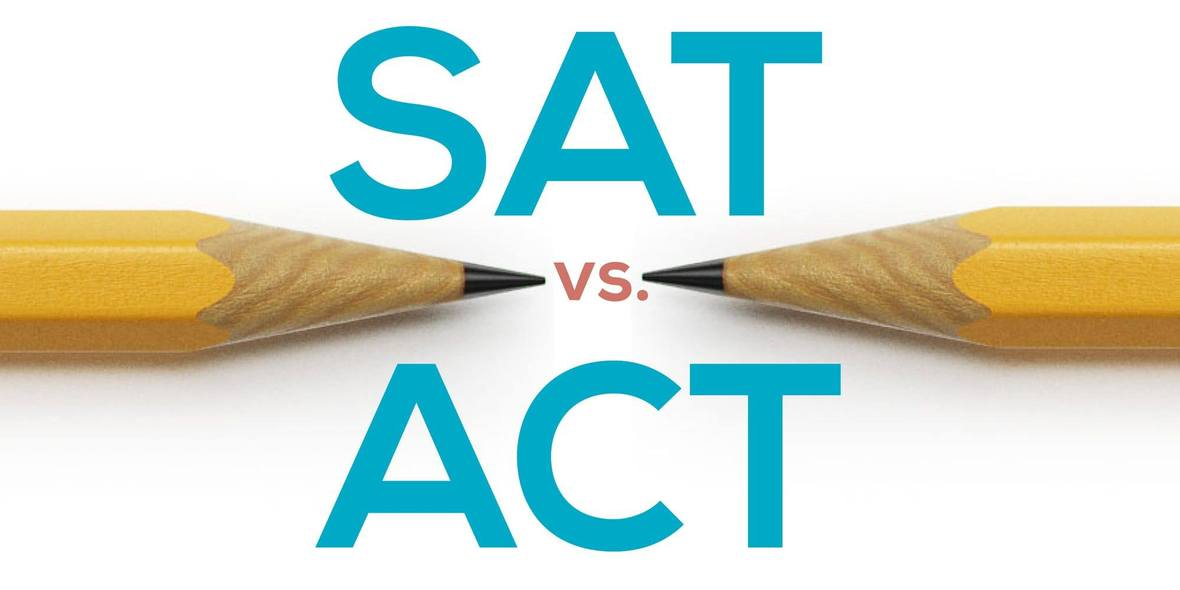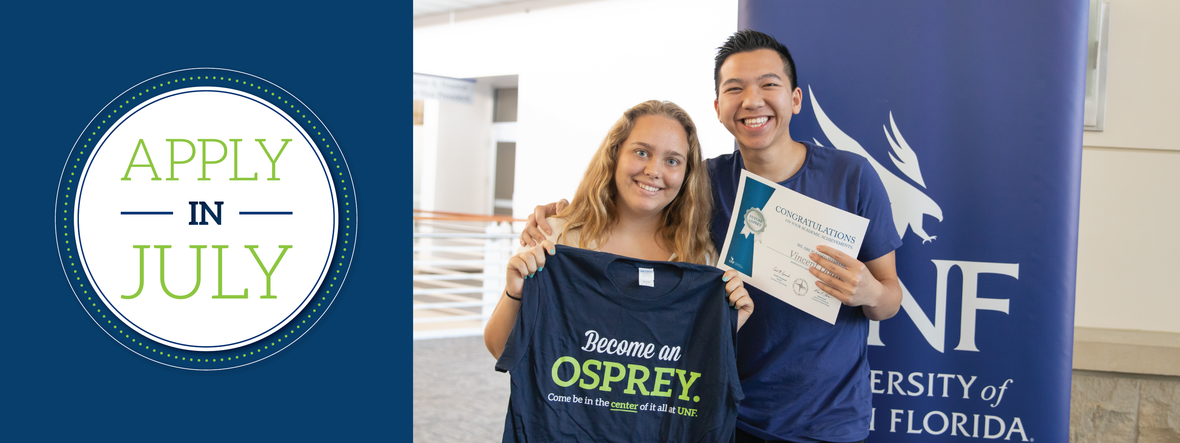# Hello, The College Map!

 table div table+table+table+table div table{width:100%;padding:0}table div table+table+table+table div table img{width:96.23%;padding:0;float:none}table div table+table+table+table div table td{width:100%;padding:0 1.88% 18px}/* styles */## The College Map office is OPEN/* styles */ The College Map re-opened on Monday, June 1st. We are adhering to the guidelines and protocol set forth by Orlando Health regarding mask wearing, hand washing, social distancing, surface cleaning, and screening (no-contact thermometer) and the Phase 2 guidelines provided by the State of Florida Health Department. Our hours will be limited to scheduled one-on-one college planning appointments. Counselors will work with their students to schedule appointments within these parameters throughout the summer. Please know that we will continue to offer virtual meetings to those students who have high risk family members and do not feel comfortable coming into the office.
 table div table+table+table+table+table+table+table+table div table{width:100%;padding:0}table div table+table+table+table+table+table+table+table div table img{width:96.23%;padding:0;float:none}table div table+table+table+table+table+table+table+table div table td{width:100%;padding:0 1.88% 18px}/* styles */## TCM Summer RemindersThe College Map will be closed every Saturday in June and July and Friday, July 3rd - Friday, July 10th

## Summer Hours and Important Dates

Saturdays in June and July:
CLOSED

Friday, July 3rd - Friday, July 10th:
CLOSED. Happy Independence Day!

Thursday, July 9th:
UNF Apply in July Event.

Rising Senior Boot Camp: Monday, August 3rd - Thursday, August 6th from 10am-6pm.

 table div table+table+table+table+table+table+table+table+table+table+table+table div table{width:100%;padding:0}table div table+table+table+table+table+table+table+table+table+table+table+table div table img{width:96.23%;padding:0;float:none}table div table+table+table+table+table+table+table+table+table+table+table+table div table td{width:100%;padding:0 1.88% 18px}/* styles */## August SAT Subject Tests/* styles */ Due to the cancelation of the May and June SAT exams, many students were not able to sit for their SAT Subject Tests. Please remember to re-schedule those exams for August! Subject Tests will not be offered in September. Subject tests are subject specific college admissions exams. They are designed by the same company (College Board) that publishes the SAT and AP exams. There are twenty different subject tests available in the areas of English, Math, Science, and foreign language. They are one hour long multiple choice tests that are given on the dates that the SAT is given- but, not all twenty tests are offered on the same date. Here is a list of updated colleges requiring SAT Subject Tests.
 table div table+table+table+table+table+table+table+table+table+table+table+table+table+table+table+table div table{width:100%;padding:0}table div table+table+table+table+table+table+table+table+table+table+table+table+table+table+table+table div table img{width:96.23%;padding:0;float:none}table div table+table+table+table+table+table+table+table+table+table+table+table+table+table+table+table div table td{width:100%;padding:0 1.88% 18px}/* styles */## Be Intentional In Your College Search!table div table+table+table+table+table+table+table+table+table+table+table+table+table+table+table+table+table+table+table+table div table{width:100%;padding:0}table div table+table+table+table+table+table+table+table+table+table+table+table+table+table+table+table+table+table+table+table div table img{width:96.23%;padding:0;float:none}table div table+table+table+table+table+table+table+table+table+table+table+table+table+table+table+table+table+table+table+table div table td{width:100%;padding:0 1.88% 18px}/* styles */## Class of 2021 Checklist!table div table+table+table+table+table+table+table+table+table+table+table+table+table+table+table+table+table+table+table+table+table+table+table+table+table div table{width:100%;padding:0}table div table+table+table+table+table+table+table+table+table+table+table+table+table+table+table+table+table+table+table+table+table+table+table+table+table div table img{width:96.23%;padding:0;float:none}table div table+table+table+table+table+table+table+table+table+table+table+table+table+table+table+table+table+table+table+table+table+table+table+table+table div table td{width:100%;padding:0 1.88% 18px}/* styles */## Transcript Orders/* styles */ Seniors in our College Planning program: Your junior year grades should officially be finalized and processed on your transcript by mid June. Please order an unofficial copy from your school. We need to verify the transcript for accuracy over the summer to give a head-start on any possible corrections that need to be made. Remember: you'll be sending official transcripts to each college you apply to; it's imperative that they be accurate and updated prior to that time. Note: We will check it one final time before you send the official copies to colleges (that particular version must have your senior year schedule on it, which usually happens in September of your senior year).
 table div table+table+table+table+table+table+table+table+table+table+table+table+table+table+table+table+table+table+table+table+table+table+table+table+table+table+table+table+table div table{width:100%;padding:0}table div table+table+table+table+table+table+table+table+table+table+table+table+table+table+table+table+table+table+table+table+table+table+table+table+table+table+table+table+table div table img{width:96.23%;padding:0;float:none}table div table+table+table+table+table+table+table+table+table+table+table+table+table+table+table+table+table+table+table+table+table+table+table+table+table+table+table+table+table div table td{width:100%;padding:0 1.88% 18px}/* styles */## SAT/ACT Diagnostic Review Sessions/* styles */ Juniors (Class of 2021)...., if you recently took the ACT/SAT diagnostic assessment, please plan to attend one of the following post-diagnostic meetings and test prep planning meetings. You will receive your diagnostic results and you will learn about the next steps in your test prep journey with us. Students and parents are encouraged to attend. Please email Julie Mitchell at juliemitchell@thecollegemap.com to r.s.v.p. Please choose one of the following dates: June 16th @ 5:00 p.m. June 17th @ 5:00 p.m. June 18th @ 5:00 p.m. June 30th @ 5:00 p.m. July 1st @ 5:00 p.m. July 2nd @ 5:00 p.m. July 14th @ 5:00 p.m. July 15th @ 5:00 p.m. July 16th @ 5:00 p.m.
 table div table+table+table+table+table+table+table+table+table+table+table+table+table+table+table+table+table+table+table+table+table+table+table+table+table+table+table+table+table+table+table+table+table div table{width:100%;padding:0}table div table+table+table+table+table+table+table+table+table+table+table+table+table+table+table+table+table+table+table+table+table+table+table+table+table+table+table+table+table+table+table+table+table div table img{width:96.23%;padding:0;float:none}table div table+table+table+table+table+table+table+table+table+table+table+table+table+table+table+table+table+table+table+table+table+table+table+table+table+table+table+table+table+table+table+table+table div table td{width:100%;padding:0 1.88% 18px}/* styles */## How to Apply for ACT and SAT Accommodationstable div table+table+table+table+table+table+table+table+table+table+table+table+table+table+table+table+table+table+table+table+table+table+table+table+table+table+table+table+table+table+table+table+table+table+table+table+table div table{width:100%;padding:0}table div table+table+table+table+table+table+table+table+table+table+table+table+table+table+table+table+table+table+table+table+table+table+table+table+table+table+table+table+table+table+table+table+table+table+table+table+table div table img{width:96.23%;padding:0;float:none}table div table+table+table+table+table+table+table+table+table+table+table+table+table+table+table+table+table+table+table+table+table+table+table+table+table+table+table+table+table+table+table+table+table+table+table+table+table div table td{width:100%;padding:0 1.88% 18px}/* styles */## Senior PARENT Financial Aid and Scholarship Meeting - 8/29 at 10am/* styles */ Save the Date! Our annual Financial Aid and Scholarship Meeting will be held on Saturday, August 29th at 10:00am. We strongly recommend attending this meeting and we have specifically scheduled it prior to FAFSA opening. This is your chance to learn more about applying for the various forms of financial aid! This event is only for families enrolled in our College Planning services. Want to get a head-start on your scholarship search? Visit "Your Path" and click on the Financial Aid tab for some helpful links.
 table div table+table+table+table+table+table+table+table+table+table+table+table+table+table+table+table+table+table+table+table+table+table+table+table+table+table+table+table+table+table+table+table+table+table+table+table+table+table+table+table+table div table{width:100%;padding:0}table div table+table+table+table+table+table+table+table+table+table+table+table+table+table+table+table+table+table+table+table+table+table+table+table+table+table+table+table+table+table+table+table+table+table+table+table+table+table+table+table+table div table img{width:96.23%;padding:0;float:none}table div table+table+table+table+table+table+table+table+table+table+table+table+table+table+table+table+table+table+table+table+table+table+table+table+table+table+table+table+table+table+table+table+table+table+table+table+table+table+table+table+table div table td{width:100%;padding:0 1.88% 18px}/* styles */## Apply in July to UNFtable div table+table+table+table+table+table+table+table+table+table+table+table+table+table+table+table+table+table+table+table+table+table+table+table+table+table+table+table+table+table+table+table+table+table+table+table+table+table+table+table+table+table+table+table+table div table{width:100%;padding:0}table div table+table+table+table+table+table+table+table+table+table+table+table+table+table+table+table+table+table+table+table+table+table+table+table+table+table+table+table+table+table+table+table+table+table+table+table+table+table+table+table+table+table+table+table+table div table img{width:96.23%;padding:0;float:none}table div table+table+table+table+table+table+table+table+table+table+table+table+table+table+table+table+table+table+table+table+table+table+table+table+table+table+table+table+table+table+table+table+table+table+table+table+table+table+table+table+table+table+table+table+table div table td{width:100%;padding:0 1.88% 18px}/* styles */## COVID-19 Question on 2020-2021 Common App/* styles */ The COVID-19 pandemic has disrupted the lives and postsecondary plans for many students. We want to reduce anxiety for applicants affected by these events and provide them with a way to share their experience with colleges and universities. Next year, on the 2020-2021 application, Common App will provide students who need it with a dedicated space to elaborate on the impact of the pandemic, both personally and academically. We want to provide colleges with the information they need, with the goal of having students answer COVID-19 questions only once while using the rest of the application as they would have before to share their interests and perspectives beyond COVID-19. Below is the question applicants will see: Community disruptions such as COVID-19 and natural disasters can have deep and long-lasting impacts. If you need it, this space is yours to describe those impacts. Colleges care about the effects on your health and well-being, safety, family circumstances, future plans, and education, including access to reliable technology and quiet study spaces. Do you wish to share anything on this topic? Y/N Please use this space to describe how these events have impacted you. The question will be optional and will appear in the Additional Information section of the application.The response length will be limited to 250 words. The question will be accompanied by a more detailed FAQ to help students consider the kinds of impacts they may wish to report, including illness and loss, housing and employment disruptions, and shifting family obligations.
 table div table+table+table+table+table+table+table+table+table+table+table+table+table+table+table+table+table+table+table+table+table+table+table+table+table+table+table+table+table+table+table+table+table+table+table+table+table+table+table+table+table+table+table+table+table+table+table+table+table div table{width:100%;padding:0}table div table+table+table+table+table+table+table+table+table+table+table+table+table+table+table+table+table+table+table+table+table+table+table+table+table+table+table+table+table+table+table+table+table+table+table+table+table+table+table+table+table+table+table+table+table+table+table+table+table div table img{width:96.23%;padding:0;float:none}table div table+table+table+table+table+table+table+table+table+table+table+table+table+table+table+table+table+table+table+table+table+table+table+table+table+table+table+table+table+table+table+table+table+table+table+table+table+table+table+table+table+table+table+table+table+table+table+table+table div table td{width:100%;padding:0 1.88% 18px}/* styles */## Virtual College Tours/* styles */ Below is a great link to over 900 colleges/universities with links to virtual tours and unique features of each school all in one place. This is a great one-stop shop for our students, specifically our juniors who may not be able to visit colleges in person in the coming months and to seniors still trying to make a decision. Please click HERE Road Trip to College runs through the month of June 2020 https://www.roadtriptocollege.org/
 table div table+table+table+table+table+table+table+table+table+table+table+table+table+table+table+table+table+table+table+table+table+table+table+table+table+table+table+table+table+table+table+table+table+table+table+table+table+table+table+table+table+table+table+table+table+table+table+table+table+table+table+table+table div table{width:100%;padding:0}table div table+table+table+table+table+table+table+table+table+table+table+table+table+table+table+table+table+table+table+table+table+table+table+table+table+table+table+table+table+table+table+table+table+table+table+table+table+table+table+table+table+table+table+table+table+table+table+table+table+table+table+table+table div table img{width:96.23%;padding:0;float:none}table div table+table+table+table+table+table+table+table+table+table+table+table+table+table+table+table+table+table+table+table+table+table+table+table+table+table+table+table+table+table+table+table+table+table+table+table+table+table+table+table+table+table+table+table+table+table+table+table+table+table+table+table+table div table td{width:100%;padding:0 1.88% 18px}/* styles */## Foreign Language Online Resource/* styles */ Here is a great resource for students struggling with foreign languages online.
 table div table+table+table+table+table+table+table+table+table+table+table+table+table+table+table+table+table+table+table+table+table+table+table+table+table+table+table+table+table+table+table+table+table+table+table+table+table+table+table+table+table+table+table+table+table+table+table+table+table+table+table+table+table+table+table+table+table div table{width:100%;padding:0}table div table+table+table+table+table+table+table+table+table+table+table+table+table+table+table+table+table+table+table+table+table+table+table+table+table+table+table+table+table+table+table+table+table+table+table+table+table+table+table+table+table+table+table+table+table+table+table+table+table+table+table+table+table+table+table+table+table div table img{width:96.23%;padding:0;float:none}table div table+table+table+table+table+table+table+table+table+table+table+table+table+table+table+table+table+table+table+table+table+table+table+table+table+table+table+table+table+table+table+table+table+table+table+table+table+table+table+table+table+table+table+table+table+table+table+table+table+table+table+table+table+table+table+table+table div table td{width:100%;padding:0 1.88% 18px}/* styles */## Bright Futures REMINDER!/* styles */ Seniors, please make sure you submit a completed Florida Financial Aid Application (FFAA) in order to be considered for State Scholarship & Grant Programs. Seniors, please Create a Student Account. After logging into your account, you may proceed to complete the FFAA. Even IF you are not attending an in-state Florida school, we highly recommend every senior complete their Bright Futures application. Florida graduates have two years to enact their scholarship money. The Bright Futures link can answer all of your questions:
 table div table+table+table+table+table+table+table+table+table+table+table+table+table+table+table+table+table+table+table+table+table+table+table+table+table+table+table+table+table+table+table+table+table+table+table+table+table+table+table+table+table+table+table+table+table+table+table+table+table+table+table+table+table+table+table+table+table+table+table+table+table div table{width:100%;padding:0}table div table+table+table+table+table+table+table+table+table+table+table+table+table+table+table+table+table+table+table+table+table+table+table+table+table+table+table+table+table+table+table+table+table+table+table+table+table+table+table+table+table+table+table+table+table+table+table+table+table+table+table+table+table+table+table+table+table+table+table+table+table div table img{width:96.23%;padding:0;float:none}table div table+table+table+table+table+table+table+table+table+table+table+table+table+table+table+table+table+table+table+table+table+table+table+table+table+table+table+table+table+table+table+table+table+table+table+table+table+table+table+table+table+table+table+table+table+table+table+table+table+table+table+table+table+table+table+table+table+table+table+table+table div table td{width:100%;padding:0 1.88% 18px}/* styles */## Monthly Scholarships/* styles */ There are thousands of scholarships out there. Below you will find many scholarships, most with general eligibility requirements. Click here for DoSomething.org Monthly Scholarships! Click here for Monthly Scholarships!
 table div table+table+table+table+table+table+table+table+table+table+table+table+table+table+table+table+table+table+table+table+table+table+table+table+table+table+table+table+table+table+table+table+table+table+table+table+table+table+table+table+table+table+table+table+table+table+table+table+table+table+table+table+table+table+table+table+table+table+table+table+table+table+table+table+table div table{width:100%;padding:0}table div table+table+table+table+table+table+table+table+table+table+table+table+table+table+table+table+table+table+table+table+table+table+table+table+table+table+table+table+table+table+table+table+table+table+table+table+table+table+table+table+table+table+table+table+table+table+table+table+table+table+table+table+table+table+table+table+table+table+table+table+table+table+table+table+table div table img{width:96.23%;padding:0;float:none}table div table+table+table+table+table+table+table+table+table+table+table+table+table+table+table+table+table+table+table+table+table+table+table+table+table+table+table+table+table+table+table+table+table+table+table+table+table+table+table+table+table+table+table+table+table+table+table+table+table+table+table+table+table+table+table+table+table+table+table+table+table+table+table+table+table div table td{width:100%;padding:0 1.88% 18px}/* styles */